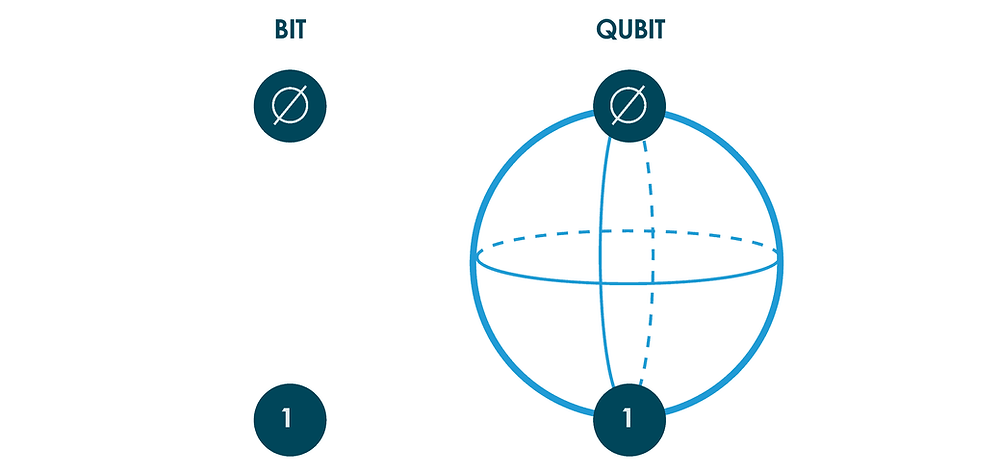Search

# What are Qubits? (03)

Updated: May 15The basic unit of classical computing is the bit. Bits can be represented by anything that can be in one of two possible states. The standard example is an electrical switch that can be either on or off. The basic unit of quantum computing is the Qubit. This can be represented by the spin of an electron or the polarization of a photon, but the properties of spin and polarization are not nearly as familiar to us as a switch being in the on or off position.

All computations involve inputting data, manipulating it according to certain rules, and then outputting the final answer. For classical computations, the bit is the basic unit of data, whereas, for quantum computations, this unit is the quantum bit—usually shortened to the qubit.

What are the main differences between a classical Bit and a Qubit?

A qubit, like a bit, includes two alternatives, but—quite unlike a bit—it can also be in a combination of these two states i.e., the state of a bit can only be either 0 or 1, the general state of a qubit according to quantum mechanics can be a coherent superposition of both.

Moreover, whereas a measurement of a classical bit would not disturb its state, a measurement of a qubit would destroy its coherence and irrevocably disturb the superposition state. It is possible to fully encode one bit in one qubit. However, a qubit can hold more information, e.g., up to two bits using superdense coding!

Qubits are more deeper than this, but we would not go far; still, if you wish to learn click here